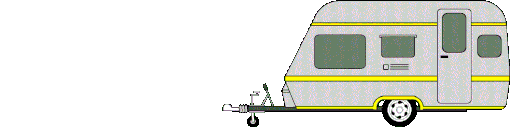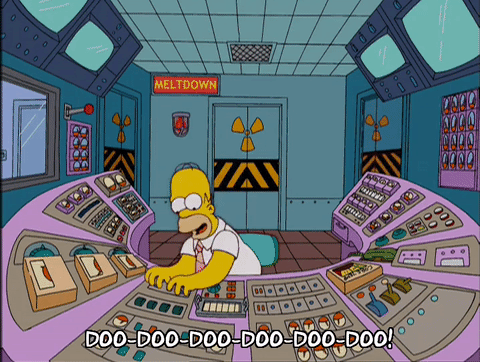# Oefening C, CK, CC, K or KK

Exercise

Fill in the correct gaps, then press "score - antwoorden" to check your answers.

1.

What is the correct spelling?

2.

What is the correct spelling?

3.4.

What is the correct spelling?

5.

What is the correct spelling?

6.

What is the correct spelling?7.

What is the correct spelling?

8.

What is the correct spelling?

9.

What is the correct spelling?10.

What is the correct spelling?

11.

What is the correct spelling?

12.

What is the correct spelling?13.

What is the correct spelling?

14.

What is the correct spelling?

15.

What is the correct spelling?16.

What is the correct spelling?

17.

What is the correct spelling?

18.

What is the correct spelling?19.

What is the correct spelling?

20.

What is the correct spelling?